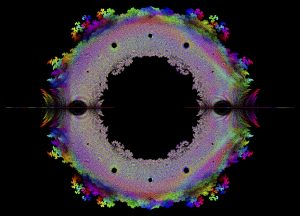# Math Cartography

Date started: Sept 2017

###Abstract

Our systems of study are families of self-similar sets parameterized by one complex variable. The internal structure of these self-similar sets is illustrated by what we call complex trees. The main advantage of introducing the notion of complex tree is that it gives us a novel way to illustrate and study the parameter spaces in$\mathbb{C}$ of families of self-similar sets. Not all parameter space maps are used for the same purpose. Some maps are designed to highlight the boundary between two regions. Others may show a particular watershed of related polynomial roots, or they may show the hierarchical distribution of roots, densities,$b$-ary entropy, or other things over a particular area of the parameter space of the family of study. Which of these maps would you like to explore, improve, and generalize?

### Media

The connectivity locus$M$ for 2-gon complex trees$T\{c,-c\}$ turns out to be the closure of all roots (contained in the unit disk) of polynomials with coefficients 1, -1, and 0, starting always with 1. The map below shows all roots of such polynomials up to degree 12. The radius and color coding of each algebraic number comes from the degree$m$ of the lowest degree polynomial who has it as a root.

If we select only roots of polynomials with coefficients equal to 1 or -1 then we obtain a subset of M which is tightly connected to the Thurston Set introduced in Bill Thurston’s last paper.

From these tip-to-tip equivalence relations we can jump to a pair of new families of connected self-similar sets: